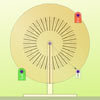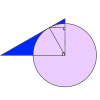# Search by Topic

#### Resources tagged with Sine, cosine, tangent similar to Quaternions and Rotations:

Filter by: Content type:
Age range:
Challenge level:

### There are 59 results

Broad Topics > Pythagoras and Trigonometry > Sine, cosine, tangent### 8 Methods for Three by One

##### Age 14 to 18 Challenge Level:

This problem in geometry has been solved in no less than EIGHT ways by a pair of students. How would you solve it? How many of their solutions can you follow? How are they the same or different?. . . .### Where Is the Dot?

##### Age 14 to 16 Challenge Level:

A dot starts at the point (1,0) and turns anticlockwise. Can you estimate the height of the dot after it has turned through 45 degrees? Can you calculate its height?### Pythagoras on a Sphere

##### Age 16 to 18 Challenge Level:

Prove Pythagoras' Theorem for right-angled spherical triangles.### Farhan's Poor Square

##### Age 14 to 16 Challenge Level:

From the measurements and the clue given find the area of the square that is not covered by the triangle and the circle.### Three by One

##### Age 16 to 18 Challenge Level:

There are many different methods to solve this geometrical problem - how many can you find?### Sine and Cosine for Connected Angles

##### Age 14 to 16 Challenge Level:

The length AM can be calculated using trigonometry in two different ways. Create this pair of equivalent calculations for different peg boards, notice a general result, and account for it.##### Age 14 to 16 Challenge Level:

If you were to set the X weight to 2 what do you think the angle might be?### History of Trigonometry - Part 3

##### Age 11 to 18

The third of three articles on the History of Trigonometry.### History of Trigonometry - Part 2

##### Age 11 to 18

The second of three articles on the History of Trigonometry.### Diagonals for Area

##### Age 16 to 18 Challenge Level:

Can you prove this formula for finding the area of a quadrilateral from its diagonals?### The History of Trigonometry- Part 1

##### Age 11 to 18

The first of three articles on the History of Trigonometry. This takes us from the Egyptians to early work on trigonometry in China.### Trig Reps

##### Age 16 to 18 Challenge Level:

Can you deduce the familiar properties of the sine and cosine functions starting from these three different mathematical representations?##### Age 14 to 16 Challenge Level:

The sides of a triangle are 25, 39 and 40 units of length. Find the diameter of the circumscribed circle.### Round and Round a Circle

##### Age 14 to 16 Challenge Level:

Can you explain what is happening and account for the values being displayed?### Sine and Cosine

##### Age 14 to 16 Challenge Level:

The sine of an angle is equal to the cosine of its complement. Can you explain why and does this rule extend beyond angles of 90 degrees?### Shape and Territory

##### Age 16 to 18 Challenge Level:

If for any triangle ABC tan(A - B) + tan(B - C) + tan(C - A) = 0 what can you say about the triangle?### Strange Rectangle 2

##### Age 16 to 18 Challenge Level:

Find the exact values of some trig. ratios from this rectangle in which a cyclic quadrilateral cuts off four right angled triangles.### Why Stop at Three by One

##### Age 16 to 18

Beautiful mathematics. Two 18 year old students gave eight different proofs of one result then generalised it from the 3 by 1 case to the n by 1 case and proved the general result.### Octa-flower

##### Age 16 to 18 Challenge Level:

Join some regular octahedra, face touching face and one vertex of each meeting at a point. How many octahedra can you fit around this point?### A Scale for the Solar System

##### Age 14 to 16 Challenge Level:

The Earth is further from the Sun than Venus, but how much further? Twice as far? Ten times?### Ball Bearings

##### Age 16 to 18 Challenge Level:

If a is the radius of the axle, b the radius of each ball-bearing, and c the radius of the hub, why does the number of ball bearings n determine the ratio c/a? Find a formula for c/a in terms of n.### Geometric Trig

##### Age 16 to 18 Short Challenge Level:

Trigonometry, circles and triangles combine in this short challenge.### Degree Ceremony

##### Age 16 to 18 Challenge Level:

What does Pythagoras' Theorem tell you about these angles: 90°, (45+x)° and (45-x)° in a triangle?### Screen Shot

##### Age 14 to 16 Challenge Level:

A moveable screen slides along a mirrored corridor towards a centrally placed light source. A ray of light from that source is directed towards a wall of the corridor, which it strikes at 45 degrees. . . .##### Age 11 to 18

Logo helps us to understand gradients of lines and why Muggles Magic is not magic but mathematics. See the problem Muggles magic.### Orbiting Billiard Balls

##### Age 14 to 16 Challenge Level:

What angle is needed for a ball to do a circuit of the billiard table and then pass through its original position?### Inscribed in a Circle

##### Age 14 to 16 Challenge Level:

The area of a square inscribed in a circle with a unit radius is, satisfyingly, 2. What is the area of a regular hexagon inscribed in a circle with a unit radius?### Circle Box

##### Age 14 to 16 Challenge Level:

It is obvious that we can fit four circles of diameter 1 unit in a square of side 2 without overlapping. What is the smallest square into which we can fit 3 circles of diameter 1 unit?### Circle Scaling

##### Age 14 to 16 Challenge Level:

Describe how to construct three circles which have areas in the ratio 1:2:3.### Round and Round

##### Age 14 to 16 Challenge Level:

Prove that the shaded area of the semicircle is equal to the area of the inner circle.### Figure of Eight

##### Age 14 to 16 Challenge Level:

On a nine-point pegboard a band is stretched over 4 pegs in a "figure of 8" arrangement. How many different "figure of 8" arrangements can be made ?### Spokes

##### Age 16 to 18 Challenge Level:

Draw three equal line segments in a unit circle to divide the circle into four parts of equal area.### Moving Squares

##### Age 14 to 16 Challenge Level:

How can you represent the curvature of a cylinder on a flat piece of paper?### Dodecawhat

##### Age 14 to 16 Challenge Level:

Follow instructions to fold sheets of A4 paper into pentagons and assemble them to form a dodecahedron. Calculate the error in the angle of the not perfectly regular pentagons you make.### Squ-areas

##### Age 14 to 16 Challenge Level:

Three squares are drawn on the sides of a triangle ABC. Their areas are respectively 18 000, 20 000 and 26 000 square centimetres. If the outer vertices of the squares are joined, three more. . . .##### Age 14 to 16 Challenge Level:

In this problem we are faced with an apparently easy area problem, but it has gone horribly wrong! What happened?### The Dodecahedron

##### Age 16 to 18 Challenge Level:

What are the shortest distances between the centres of opposite faces of a regular solid dodecahedron on the surface and through the middle of the dodecahedron?### So Big

##### Age 16 to 18 Challenge Level:

One side of a triangle is divided into segments of length a and b by the inscribed circle, with radius r. Prove that the area is: abr(a+b)/ab-r^2### Gold Again

##### Age 16 to 18 Challenge Level:

Without using a calculator, computer or tables find the exact values of cos36cos72 and also cos36 - cos72.### Cosines Rule

##### Age 14 to 16 Challenge Level:

Three points A, B and C lie in this order on a line, and P is any point in the plane. Use the Cosine Rule to prove the following statement.### 30-60-90 Polypuzzle

##### Age 16 to 18 Challenge Level:

Re-arrange the pieces of the puzzle to form a rectangle and then to form an equilateral triangle. Calculate the angles and lengths.### Eight Ratios

##### Age 14 to 16 Challenge Level:

Two perpendicular lines lie across each other and the end points are joined to form a quadrilateral. Eight ratios are defined, three are given but five need to be found.### Over the Pole

##### Age 16 to 18 Challenge Level:

Two places are diametrically opposite each other on the same line of latitude. Compare the distances between them travelling along the line of latitude and travelling over the nearest pole.### Raising the Roof

##### Age 14 to 16 Challenge Level:

How far should the roof overhang to shade windows from the mid-day sun?### Flight Path

##### Age 16 to 18 Challenge Level:

Use simple trigonometry to calculate the distance along the flight path from London to Sydney.### Belt

##### Age 16 to 18 Challenge Level:

A belt of thin wire, length L, binds together two cylindrical welding rods, whose radii are R and r, by passing all the way around them both. Find L in terms of R and r.### Trigonometric Protractor

##### Age 14 to 16 Challenge Level:

An environment that simulates a protractor carrying a right- angled triangle of unit hypotenuse.### Logosquares

##### Age 16 to 18 Challenge Level:

Ten squares form regular rings either with adjacent or opposite vertices touching. Calculate the inner and outer radii of the rings that surround the squares.### Six Discs

##### Age 14 to 16 Challenge Level:

Six circular discs are packed in different-shaped boxes so that the discs touch their neighbours and the sides of the box. Can you put the boxes in order according to the areas of their bases?### Far Horizon

##### Age 14 to 16 Challenge Level:

An observer is on top of a lighthouse. How far from the foot of the lighthouse is the horizon that the observer can see?